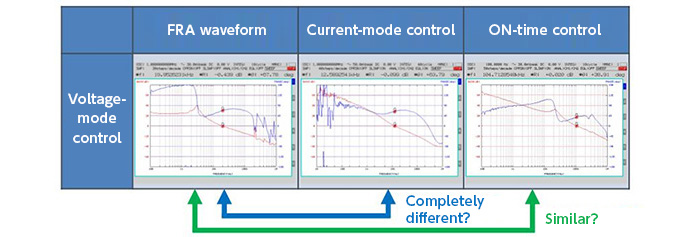Technical Information Site of Power Supply Design

2016.09.08 Transfer Function

Introduction

DC/DC Converters: Sharing of Transfer Functions among Control Systems

A transfer function is a function that represents the relation between the input and the output of a system, converting the input into the output. In control engineering, transfer functions are used as means for evaluating the behavior and stability of a system. Of course, power supplies, which are the main theme of this website, have transfer functions, and by determining the transfer function as part of power supply design, the response characteristics and stability can be evaluated. In this "series on transfer functions", we develop a discussion of, among other things, transfer functions for DC/DC converters and approaches to deriving transfer functions. There will be a few somewhat complicated equations, but a firm understanding of transfer functions is extremely worthwhile.

Here, however, the topic is determining the transfer function of a DC/DC converter, and we shall, as the title indicates, be addressing the issue of a "common approach to transfer functions for control systems".

In order to derive the transfer function for a DC/DC converter, the switching operation must be time-averaged over a cycle and then approximated as a linear operation. Moreover, the derived transfer function will differ depending on the type of voltage conversion, whether stepping down, stepping up, or stepping up and down, as well as on the control method, such as voltage mode, current mode, and fixed on-time hysteresis control (also called ripple control, comparator control.). In addition, various approaches are used depending on the mode, and consequently things get quite troublesome.

Hence on the assumption that such varied transfer functions could be summarized in a single unified approach, we decided, when addressing the derivation of transfer functions for DC/DC converters, to make a "common approach" our theme.

The following is a more specific discussion. The image below shows, from left to right, waveforms measured by a FRA (Frequency Response Analyzer) for "voltage mode control", "current mode control", and "fixed on-time hysteresis control" of a step-down converter. Upon comparing the characteristics of such different control methods side-by-side, I think one sees that there are completely different parts, and quite similar parts. It seems that these transfer functions could be derived using a single method based on a common concept and approach, and then represent the different characteristics as a single function on a common platform.Here, the following two points should be born in mind in what follows.

1. Processes for transfer function derivation are based on a mathematical approach.
In the process of deriving a transfer function, such mathematical methods as state averaging and matrices are used to confront the transfer function head-on. However, ultimately we want to be able to derive the transfer function conceptually, without using such methods.

2. A unified approach to different control methods is taken.
It is assumed that separate approaches are not taken to different modes, such as step-down or step-up mode, and voltage or current mode. While using the same approach, the features of each mode are incorporated to derive the transfer function.

Finally, we hope it will be understood that even if DC/DC converter control modes are different, the core remains the same. The goal is to derive a transfer function that maintains the generality and uniformity of a function, by capturing the common parts and the features of different modes.